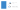Yield definition

What is a yield?

A yield is the income earned from an investment, most often in the form of interest or dividend payments. A yield is one of the ways in which an investment can earn a trader money, with the other being the sale of the asset.

Most often, a yield will be expressed as a yearly percentage of either the value of the original investment, or of its current market value.

Yield calculation and formula

The calculation for yield differs depending on the type of yield. The common formula is income (eg from dividends or interest payments) divided by investment value. This can then be multiplied by 100 to get a percentage figure.Types of yield in finance

Dividend yield

Dividend yield compares a company’s annual dividends to its share price. It is a popular method used by dividend investors, who prefer to take advantage of regular dividend payments. To calculate dividend yield, you’d divide the company’s annual dividend by its current share price.

For example, say shares of company XYZ are trading at £5 and it pays a dividend of 20p, the dividend yield is 4% (20p/£5 = 0.04).

Stock yield

Stock yield measures the growth of an investment. It is a popular method among value investors, who look for stocks with strong growth potential.

There are two ways to measure stock yield – stock return and rate of return. Return is usually given as an amount of the current share price, rather than a percentage. For example, if the share price grows from £5 to £6, the stock yield is 100p (£6 - £5 = 100p).

The rate of return is given as a percentage; calculated by subtracting the starting value of the investment from its final value and then dividing the figure by the starting value. It is then multiplied by 100 to get the percentage value. For example, if the starting value is £5000 and the final value is £5650, the rate of return is 13% ([£5650 - £5000]/£5000 x 100).

Bond yield

Bond yield measures the return an investor realises on a bond. It can be calculated in multiple ways but is commonly given as either a percentage of the bond price when it is issued or a percentage of the current price of the bond. To calculate a bond’s yield using these methods, you’d divide the interest on the bond by your chosen price, and then multiply it by 100.

For example, a bond with the value of £5000 that pays annual interest of £100 would have a yield of 2% ([£100/£5000] x 100 = 2).

Discover how to trade with IG Academy, using our series of interactive courses, webinars and seminars.

A - B - C - D - E - F - G - H - I - L - M - N - O - P - Q - R - S - T - U - V - W - Y

See all glossary trading terms